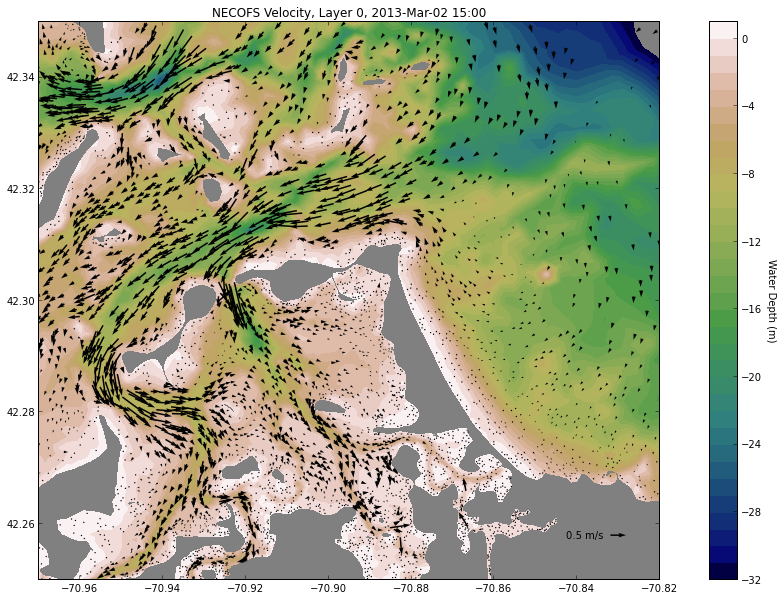## Access data from the NECOFS (New England Coastal Ocean Forecast System) via OPeNDAP¶

Demonstration using the NetCDF4-Python library to access velocity data from a triangular grid ocean model (FVCOM) via OPeNDAP, specifying the desired URL, time, layer and lat/lon region of interest. The resulting plot of forecast velocity vectors over color-shaded bathymetry is useful for a variety of recreational and scientific purposes.

NECOFS (Northeastern Coastal Ocean Forecast System) is run by groups at the University of Massachusetts Dartmouth and the Woods Hole Oceanographic Institution, led by Drs. C. Chen, R. C. Beardsley, G. Cowles and B. Rothschild. Funding is provided to run the model by the NOAA-led Integrated Ocean Observing System and the State of Massachusetts.

NECOFS is a coupled numerical model that uses nested weather models, a coastal ocean circulation model, and a wave model. The ocean model is a volume-mesh model with horizontal resolution that is finer in complicated regions. It is layered (not depth-averaged) and includes the effects of tides, winds, and varying water densities caused by temperature and salinity changes.

In :
from pylab import *
import matplotlib.tri as Tri
import netCDF4
import datetime as dt

In :
# DAP Data URL
url = 'http://www.smast.umassd.edu:8080/thredds/dodsC/FVCOM/NECOFS/Forecasts/NECOFS_FVCOM_OCEAN_MASSBAY_FORECAST.nc'
# Open DAP
nc = netCDF4.Dataset(url)
nc.variables.keys()

Out:
[u'x',
u'y',
u'lon',
u'lat',
u'xc',
u'yc',
u'lonc',
u'latc',
u'siglay',
u'siglev',
u'h',
u'nv',
u'time',
u'Times',
u'zeta',
u'nbe',
u'aw0',
u'awx',
u'awy',
u'u',
u'v',
u'ww',
u'temp',
u'salinity']
In :
# take a look at the "metadata" for the variable "u"
print nc.variables['u']

<type 'netCDF4.Variable'>
float32 u(u'time', u'siglay', u'nele')
long_name: Eastward Water Velocity
units: meters s-1
grid: fvcom_grid
type: data
unlimited dimensions = (u'time',)
current size = (145, 10, 165095)


In :
# Desired time for snapshot
# ....right now (or some number of hours from now) ...
start = dt.datetime.utcnow() + dt.timedelta(hours=0)
# ... or specific time (UTC)
start = dt.datetime(2013,3,2,15,0,0)

In :
# Get desired time step
time_var = nc.variables['time']
itime = netCDF4.date2index(start,time_var,select='nearest')

# Get lon,lat coordinates for nodes (depth)
lat = nc.variables['lat'][:]
lon = nc.variables['lon'][:]
# Get lon,lat coordinates for cell centers (depth)
latc = nc.variables['latc'][:]
lonc = nc.variables['lonc'][:]
# Get Connectivity array
nv = nc.variables['nv'][:].T - 1
# Get depth
h = nc.variables['h'][:]  # depth

In :
dtime = netCDF4.num2date(time_var[itime],time_var.units)
daystr = dtime.strftime('%Y-%b-%d %H:%M')
print daystr

2013-Mar-02 15:00

In :
tri = Tri.Triangulation(lon,lat, triangles=nv)

In :
# get current at layer [0 = surface, -1 = bottom]
ilayer = 0
u = nc.variables['u'][itime, ilayer, :]
v = nc.variables['v'][itime, ilayer, :]

In :
levels=arange(-32,2,1)   # depth contours to plot
ax= [-70.97, -70.82, 42.25, 42.35] # region to plot

In :
# find velocity points in bounding box
ind = argwhere((lonc >= ax) & (lonc <= ax) & (latc >= ax) & (latc <= ax))

In :
subsample=3
np.random.shuffle(ind)
Nvec = int(len(ind) / subsample)
idv = ind[:Nvec]

In :
# tricontourf plot of water depth with vectors on top
fig1 = figure(figsize=(18,10))

<matplotlib.text.Text at 0x49eab90>